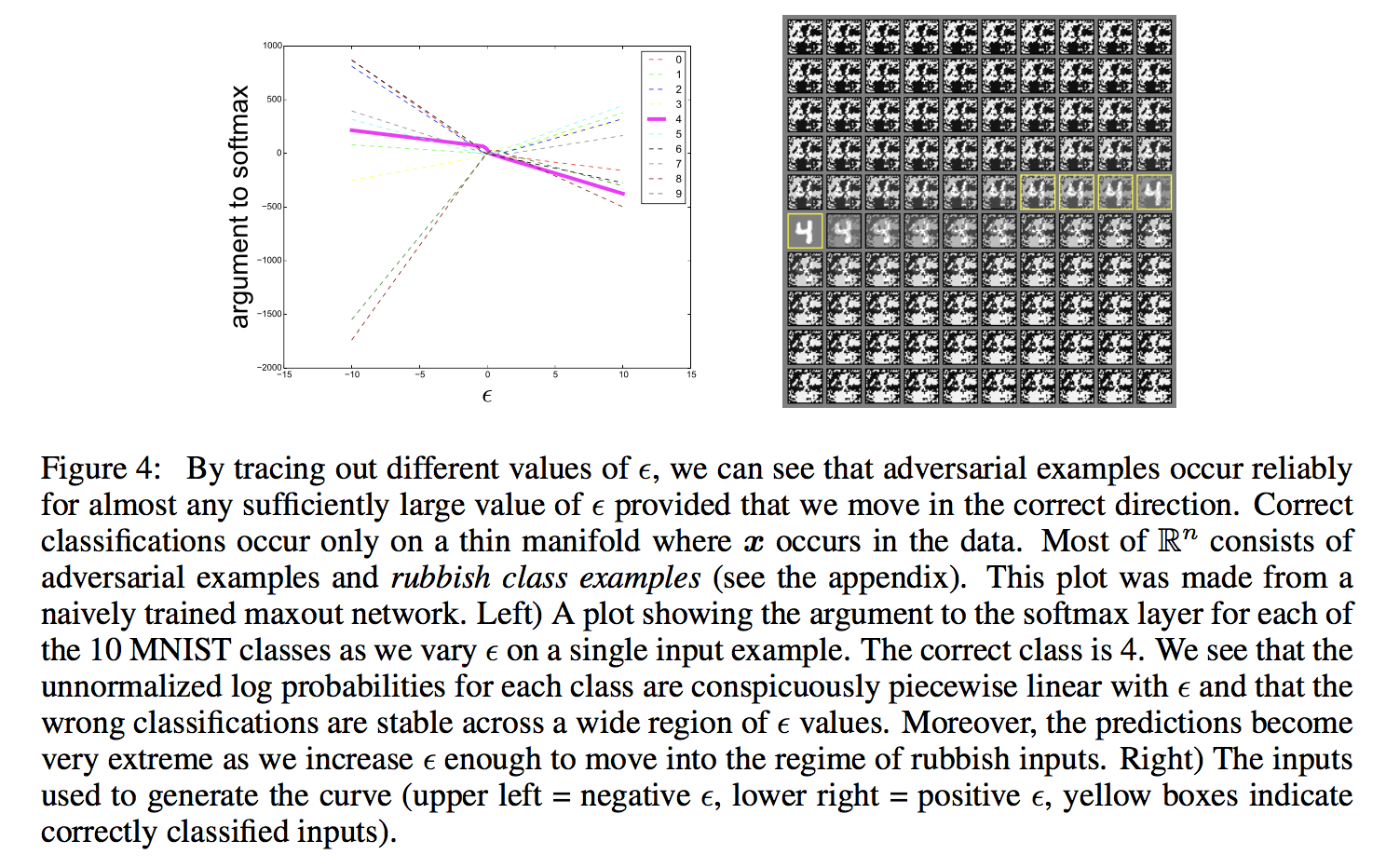# 关于Explaining and harnessing adversarial examples的理解

之前在这篇论文[Intriguing properties of neural networks]中，发现了关于神经网络的一个有趣的性质，即在原样本点上加上一些针对性的但是不易察觉的扰动，就会很容易的将神经网络分类错误，并且可能以很高的置信度输出。基于原文章中L-BFGS-B不精确求解非凸问题的速度较慢，以及很多人将这个性质的原因归结为深层神经网络的高度非线性以及过拟合，Goodfellow 用这篇论文给出了不同的看法。
他认为，即使是线性模型，仍然也会存在对抗样本，我们记输入样本~$\mathbit{x}$$\boldsymbol{x}$~的对抗样本为：$\stackrel{\mathbf{~}}{\mathbit{x}}=\mathbit{x}+\eta$$\boldsymbol{\tilde{x}}=\boldsymbol{x}+\eta$，其中要求$\eta$$\eta$足够小：$||\eta |{|}_{\mathrm{\infty }}\le ϵ$$||\eta||_{\infty}\leq \epsilon$。我们考虑权重向量$\mathbit{w}$$\boldsymbol{w}$和对抗样本$\stackrel{\mathbf{~}}{\mathbit{x}}$$\boldsymbol{\tilde{x}}$的内积：

${\mathbit{w}}^{T}\stackrel{~}{x}={\mathbit{w}}^{T}\mathbit{x}+{\mathbit{w}}^{T}\eta$

而对于神经网络而言，很多神经网络为了节省计算上的代价，都被设计成了非常线性的形式，这使得他们更容易优化，但是这样"廉价"的网络也导致了对于对抗扰动的脆弱性。他们的实验也证明了这一点，Goodfellow直接将线性扰动加入原样本：
$\stackrel{~}{\mathbit{x}}=\mathbit{x}+ϵ\text{sign}\left({\mathrm{\nabla }}_{x}J\left(\mathbit{\theta },\mathbit{x},{y}_{true}\right)\right)$

除了生成对抗样本来攻击神经网络以外，对抗训练神经网络从而有效防止对抗样本的攻击也是一个值得考虑的问题。
我们先考虑最简单的逻辑回归模型：如果我们训练一个简单的模型来识别标记$y\in \left\{-1,+1\right\}$$y \in \{-1,+1\}$，并且$p=P\left(y=1\phantom{\rule{thinmathspace}{0ex}}|\phantom{\rule{thinmathspace}{0ex}}\mathbit{x}\right)=\frac{1}{1+{e}^{-}f}=\frac{1}{1+{e}^{-\left({\mathbit{w}}^{T}\mathbit{x}+b\right)}}$$p=P(y=1 \,|\, \boldsymbol{x})={1 \over {1+e^-f}}={1 \over {1+e^{-(\boldsymbol{w}^T\boldsymbol{x}+b)}}}$。注意这里与文中相同，选择的标记为$\left\{-1,+1\right\}$$\{-1,+1\}$并不是我们平时常用的$\left\{0,1\right\}$$\{0,1\}$，我们可以简单推导一下该情况下的损失函数：
$\begin{array}{rl}J& =-\frac{1+y}{2}\mathrm{log}P\left(y=1\phantom{\rule{thinmathspace}{0ex}}|\phantom{\rule{thinmathspace}{0ex}}\mathbit{x}\right)-\frac{1-y}{2}\mathrm{log}P\left(y=-1\phantom{\rule{thinmathspace}{0ex}}|\phantom{\rule{thinmathspace}{0ex}}\mathbit{x}\right)\\ & =-\frac{1}{2}\mathrm{log}p\left(1-p\right)-\frac{y}{2}\mathrm{log}\frac{p}{1-p}\\ & =-\frac{1}{2}\mathrm{log}{e}^{-f}+\mathrm{log}\left(1+{e}^{-f}\right)-\frac{y}{2}\mathrm{log}{e}^{f}\\ & =\frac{1}{2}\left(f-yf\right)+\mathrm{log}\left(1+{e}^{-f}\right)\\ & =\left\{\begin{array}{rl}& \mathrm{log}\left(1+{e}^{-f}\right)\phantom{\rule{1em}{0ex}}y=1\\ & f+\mathrm{log}\left(1+{e}^{-f}\right)=\mathrm{log}\left({e}^{f}\right)+\mathrm{log}\left(1+{e}^{-f}\right)=\mathrm{log}\left(1+{e}^{f}\right)\phantom{\rule{1em}{0ex}}y=-1\end{array}\\ & =\mathrm{log}\left(1+{e}^{-yf}\right)\end{array}$

${\mathrm{\nabla }}_{x}J=\frac{{e}^{-yf}}{1+{e}^{-yf}}\left(-y\right)\mathbit{w}$

$\begin{array}{rl}J& =\mathrm{log}\left(1+{e}^{-y\left({\mathbit{w}}^{T}\mathbit{x}-ϵy{\mathbit{w}}^{T}\text{sign}\left(\mathbit{w}\right)+b\right)}\right)\\ & =\mathrm{log}\left(1+{e}^{-y\left({\mathbit{w}}^{T}\mathbit{x}+b\right)+ϵ||w|{|}_{1}}\right)\end{array}$

进一步的，作者基于FGSM生成的对抗样本提出了一个高效的对抗训练方法：
$\stackrel{~}{J}\left(\mathbit{\theta },\mathbit{x},y\right)=\alpha J\left(\mathbit{\theta },\mathbit{x},y\right)+\left(1-\alpha \right)J\left(\mathbit{\theta },\mathbit{x}+ϵ\text{sign}\left({\mathrm{\nabla }}_{x}J\left(\mathbit{\theta },\mathbit{x},y\right)\right)$

对于为什么会有如此大量的对抗样本，Goodfellow的解释是，只要选择的扰动方向正确，并且有足够大的$ϵ$$\epsilon$，则产生的扰动就足够大，就会改变原先的样本标记。我们可以跟踪不同的 $ϵ$$\epsilon$值，可以发现对抗样本出现在不同的连续区域。这就解释了为什么对抗性的例子非常丰富，为什么一个分类器错误分类的例子具有相当高的被另一个分类器错误分类的可能性。${\stackrel{~}{\mathbit{x}}}_{i+1}=Cli{p}_{\mathbit{x},ϵ}\left\{{\mathbit{x}}_{i}-\alpha \text{sign}\left({\mathrm{\nabla }}_{x}J\left(\mathbit{\theta },{\mathbit{x}}_{i},{y}_{target}\right)\right\}$